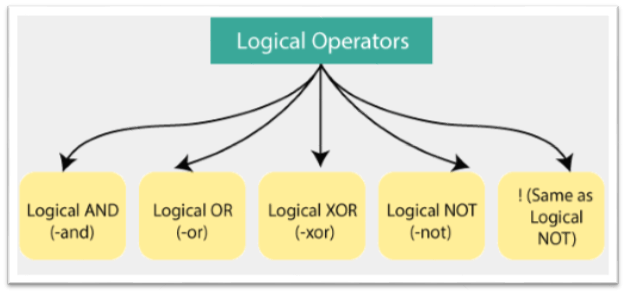# Logical Operators

The logical operators are used in PowerShell to connect expressions or statements together to form a single expression. Those expressions which contain these operators usually results in the Boolean values True or False. These operators allow us to use a single expression to test for multiple conditions.

PowerShell supports the following logical operators:1. -and (Logical AND)
2. -or (Logical OR)
3. -xor (Logical XOR)
4. -not (Logical NOT)
5. ! (Same as Logical NOT)

## -and (Logical AND)

The AND operator is the logical operator, which returns the Boolean value TRUE if both the conditions are correct in an expression.

Example: The following example describes how to use the logical AND operator:

The last command in this example displays the Boolean Value TRUE because both the conditions are correct.

## -or (Logical OR)

The OR operator is the logical operator, which returns the Boolean value TRUE if at least one of the conditions is correct in an expression.

Example: The following example describes how to use the logical OR operator:

The last command in this example displays the Boolean Value TRUE because the first condition in this statement is correct.

## -xor (Logical XOR)

The XOR operator is the logical operator, which returns the Boolean value TRUE if only one condition is correct in expression. When more than one statement is correct in expression, this operator returns the FALSE.

Example: The following example describes how to use the logical XOR operator:

The last command in this example displays the Boolean Value FALSE because, in this expression, the two conditions are correct.

## -not (Logical NOT)

The NOT operator is the logical operator that produces the opposite result of an expression. If an expression gives TRUE value, then this operator will return FALSE and vice versa.

Example: The following example describes how to use the logical NOT operator:

The last command in this example displays the Boolean Value FALSE because, in an expression, the condition is correct, but this operator turns to the opposite.

## ! (Same as Logical NOT)

This operator is same as the logical NOT operator that produces the opposite result of an expression.

Example: The following example describes how to use this operator:

The last command in this example displays the Boolean Value TRUE because, in an expression, the condition is not correct, but this operator turns the result to the opposite.

Previous articleLearn MS Word Tutorial
Next articleWhat is PowerShell cmdlet